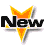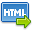Journal :   International Journal of Technology

Volume No. :   6

Issue No. :  2

Year :  2016

Pages :   99-102

ISSN Print :  2231-3907

ISSN Online :  2231-3915Registration

## An Energy Relationship in Multicomponent Convection Problem

 Address: Jyoti Prakash*, Pankaj Kumar, Shweta Manan Department of Mathematics and Statistics, Himachal Pradesh University, Summer Hill, Shimla-171005, India *Corresponding Author
DOI No: 10.5958/2231-3915.2016.00015.8

ABSTRACT:
A relationship between various energies in magnetohydrodynamic multicomponent convection problem analogous to magnetohydrodynamic thermohaline convection problem of Veronis (1965) type has been established. It is shown that the total kinetic energy associated with a disturbance exceeds the sum of its total magnetic and concentration energies in the parameter regime (Qs_1)/p^2 +(R_1 s)/(?4t?_1^2 p^4 )+(R_2 s)/(?4t?_2^2 p^4 )+?+(R_(n-1) s)/(?4t?_(n-1)^2 p^4 )=1, where Q,s,s_1,t_1,t_2,…,t_(n-1),?R_1,R?_2,…?,R?_(n-1) represent Chandrasekhar number, Prandtl number, magnetic Prandtl number, Lewis number for first concentration component, Lewis number for second concentration component, Lewis number for (n-1)th concentration component, concentration Rayleigh number for first component, concentration Rayleigh number for second component, concentration Rayleigh number for (n-1)th component respectively. Further, this result is uniformly valid for any combination of rigid or free boundaries whether perfectly conducting or insulating.
KEYWORDS:
Multicomponent convection; Chandrasekhar number; Lewis number; Prandtl number; Rayleigh number.
Cite:
Jyoti Prakash, Pankaj Kumar, Shweta Manan. An Energy Relationship in Multicomponent Convection Problem. Int. J. Tech. 2016; 6(2): 99-102.[View HTML][View PDF]

Visitor's No. :   124657